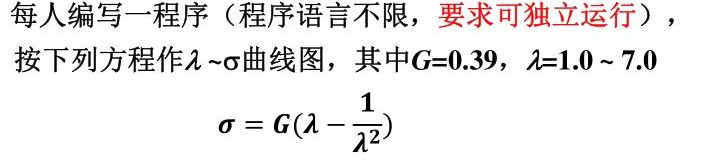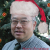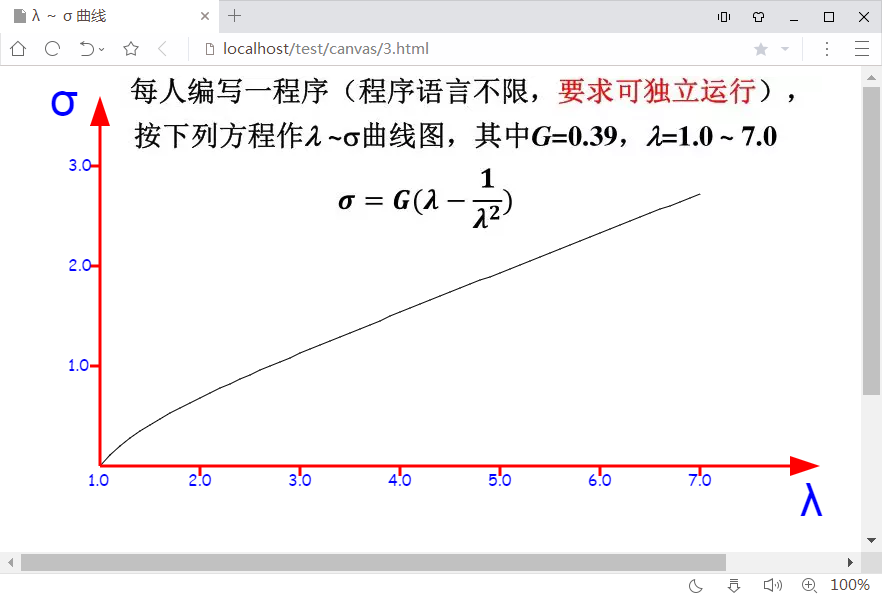# 求在线大神帮忙写个小程序

osc_47518739 发布于 11/20 21:411``````<html>
<title>λ ～ σ 曲线</title>
<style>
#myCanvas{
z-index:3;
position:absolute;
left:0px;
top:0px;
}
#img1{
z-index:1;
position:absolute;
left:120px;
top:10px;
}
</style>
<body>
<img id="img1" src="https://oscimg.oschina.net/oscnet/up-b80682b55382701db50b27f532615a908f6.png">
<canvas id="myCanvas" width="1000" height="700" >
<p>Your browserdoes not support the canvas element!</p>
</canvas>
</body>
<script>
/* 画箭头的方法：g 画笔，color 颜色，[x,y] 箭头的位置，
* d 箭头的大小， delta 箭头的取向
*/
function arrow( g, color, x, y, d, delta) {
g.save();
g.fillStyle=color;
g.translate(x,y);
g.rotate(delta*Math.PI/180);
g.beginPath();
g.moveTo( -5*d, -5*d);
g.lineTo( 5*d, -5*d);
g.lineTo( 0, 10*d);
g.closePath();
g.fill();
g.restore();
}

bigG = 0.39;
x = [];
y = [];

//计算 sigma - lambda 方程 曲线的数据，lambda [1.0，7.0] 的增量为 0.1
for (lambda = 1; lambda<=7; lambda = lambda + 0.1){
sigma = bigG*(lambda - 1/(lambda*lambda) ); //按公式计算 sigma 值
// 换算成 画布canvas 的像素数据，存入 x, y，整型数组，用来画图
x.push(lambda.toFixed(2)*100); //保留小数点后两位， 再扩大100倍,以便高分辨率画图
y.push( sigma.toFixed(2)*100);  //保留小数点后两位， 再扩大100倍,以便高分辨率画图
}

g =document.getElementById('myCanvas').getContext('2d'); //获得画笔 g
g.beginPath();
g.moveTo( x, 400- y);
for(i=1;i<x.length;i++)
g.lineTo(  x[i], 400 - y[i]);
g.stroke(); //画 出 sigma - lambda 方程 曲线

g.beginPath(); //画 X (lambda) 坐标轴
g.moveTo(100,400);
g.lineTo(800,400);
g.strokeStyle="red";
g.lineWidth="3";
g.stroke();

//标出 X (lambda) 坐标轴的刻度
g.font="16px  'Comic Sans MS', cursive";
g.fillStyle="blue";
for(i=1;i<=7;i++){
g.beginPath();
g.moveTo( 100 +i*100, 400);
g.lineTo( 100 +i*100, 410);
if(i<7)
g.stroke();
g.fillText(i + ".0", i*100-12, 420);
}
arrow(g, "red",800,400, 2, -90);

//画 Y （sigma） 坐标轴
g.beginPath();
g.moveTo(100,400);
g.lineTo(100,50);
g.strokeStyle="red";
g.lineWidth="3";
g.stroke();
arrow(g, "red",100,50, 2, 180);

for(i=1;i<4;i++){
g.beginPath();
g.moveTo( 100 , 400 -i*100 );
g.lineTo( 100-10 , 400 -i*100 );
g.stroke();
g.fillText(i + ".0", 68, 405 -i*100);
}
g.font="46px  'ARIAL', cursive";
g.fillStyle="blue";
g.fillText("λ",800,450);
g.fillText("σ",50, 50);
</script>
</html>``````